Shared Concepts and Topics
 Syntax: LSMEANS Statement

LSMEANS <model-effects> </ options> ;

LS-means can be computed for any effect in the statistical model that involves only CLASS variables. You can specify multiple effects in one LSMEANS statement or in multiple LSMEANS statements, and all LSMEANS statements must appear after the MODEL statement. If you do not specify model-effects, the options in the LSMEANS statement are applied to all suitable model effects.

As in the ESTIMATE statement, thematrix is tested for estimability; if this test fails, the procedure displays "Non-est" for the LS-means entries. Note that linear functions of LS-means, such as differences, can be estimable, even if the means themselves are not estimable. Estimability checks for differences are thus applied separately from checks for the means.

Assuming the LS-mean is estimable, the procedure constructs an approximate t test to test the null hypothesis that the associated population quantity equals zero.

Table 19.20 summarizes important options in the LSMEANS statement. All LSMEANS options are subsequently discussed in alphabetical order.

Table 19.20 Important LSMEANS Statement Options

Option

Description

Construction and Computation of LS-Means

Modifies the covariate value in computing LS-means

Computes separate margins

Requests differences of LS-means

Specifies the weighting scheme for LS-means computation as determined by the input data set

SINGULAR=

Tunes estimability checking

Degrees of Freedom and p-values

Determines the method for multiple comparison adjustment of LS-means differences

ALPHA=Determines the confidence level ()

Adjusts multiple comparison p-values further in a step-down fashion

Statistical Output

Constructs confidence limits for means and mean differences

Displays the correlation matrix of LS-means

Displays the covariance matrix of LS-means

Prints thematrix

Produces a "Lines" display for pairwise LS-means differences

Prints the LS-means

Requests ODS statistical graphics of means and mean comparisons

Specifies the seed for computations that depend on random numbers

Generalized Linear Modeling

Exponentiates and displays estimates of LS-means or LS-means differences

Computes and displays estimates and standard errors of LS-means (but not differences) on the inverse linked scale

ODDSRATIO

Reports (simple) differences of least squares means in terms of odds ratios if permitted by the link function

You can specify the following options in the LSMEANS statement after a slash (/):

specifies how denominator degrees of freedom are determined when-values and confidence limits are adjusted for multiple comparisons with the ADJUST= option. When you do not specify the ADJDFE= option or when you specify ADJDFE=SOURCE, the denominator degrees of freedom for multiplicity-adjusted results are the denominator degrees of freedom for the LS-mean effect in the "Type III Tests of Fixed Effects" table. When you specify ADJDFE=ROW, the denominator degrees of freedom for multiplicity-adjusted results correspond to the degrees of freedom that are displayed in the DF column of the "Differences of Least Squares Means" table.

The ADJDFE=ROW setting is particularly useful if you want multiplicity adjustments to take into account that denominator degrees of freedom are not constant across LS-mean differences.

In one-way models with heterogeneous variance, combining certain ADJUST= options with the ADJDFE=ROW option corresponds to particular methods of performing multiplicity adjustments in the presence of heteroscedasticity. For example, the following statements fit a heteroscedastic one-way model and perform Dunnett’s T3 method (Dunnett 1980), which is based on the studentized maximum modulus (ADJUST=SMM):

```proc glimmix;
class A;
model y = A / ddfm=satterth;
random _residual_ / group=A;
run;
```

If you combine the ADJDFE=ROW option with ADJUST=SIDAK, the multiplicity adjustment corresponds to the T2 method of Tamhane (1979), and ADJUST=TUKEY corresponds to the method of Games-Howell (Games and Howell 1976). Note that ADJUST=TUKEY gives the exact results for the case of fractional degrees of freedom in the one-way model, but it does not take into account that the degrees of freedom are subject to variability. A more conservative method, such as ADJUST=SMM, might protect the overall error rate better.

Unless the ADJUST= option is specified in the LSMEANS statement, the ADJDFE= option has no effect. The option is not supported by the procedures that perform chi-square-based inference (GENMOD, LOGISTIC, PHREG, and SURVEYLOGISTIC).

ADJUST=SMM|GT2

requests a multiple comparison adjustment for the p-values and confidence limits for the differences of LS-means. The adjusted quantities are produced in addition to the unadjusted quantities. By default, the procedure performs all pairwise differences. If you specify ADJUST=DUNNETT, the procedure analyzes all differences with a control level. If you specify ADJUST=NELSON, ANOM differences are taken. The ADJUST= option implies the DIFF option.

The BON (Bonferroni) and SIDAK adjustments involve correction factors described in Chapter 39, The GLM Procedure, and Chapter 58, The MULTTEST Procedure ; also see Westfall and Young (1993) and Westfall et al. (1999). When you specify ADJUST=TUKEY and your data are unbalanced, the procedure uses the approximation described in Kramer (1956) and identifies the adjustment as "Tukey-Kramer" in the results. Similarly, when you specify ADJUST=DUNNETT or ADJUST=NELSON and the LS-means are correlated, the procedure uses the factor-analytic covariance approximation described in Hsu (1992) and identifies the adjustment in the results as "Dunnett-Hsu" or "Nelson-Hsu," respectively. The approximation derives an approximate "effective sample sizes" for which exact critical values are computed. Computing the exact adjusted-values and critical values for unbalanced designs can be computationally intensive, in particular for ADJUST=NELSON. A simulation-based approach, as specified by the ADJUST=SIM option, while nondeterministic, can provide inferences that are sufficiently accurate in much less time. The preceding references also describe the SCHEFFE and SMM adjustments.

Nelson’s adjustment applies only to the analysis of means (Ott 1967; Nelson 1982, 1991, 1993), where LS-means are compared against an average LS-mean. It does not apply to all pairwise differences of least squares means. See the DIFF=ANOM option for more details regarding the analysis of means with the procedure.

The SIMULATE adjustment computes adjusted p-values and confidence limits from the simulated distribution of the maximum or maximum absolute value of a multivariate t random vector. All covariance parameters, except the residual scale parameter, are fixed at their estimated values throughout the simulation, potentially resulting in some underdispersion. The simulation estimates, the true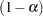th quantile, whereis the confidence coefficient. The defaultis 0.05, and you can change this value with the ALPHA= option in the LSMEANS statement.

The number of samples is set so that the tail area for the simulatedis withinofwith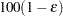% confidence. In equation form,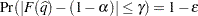whereis the simulatedandis the true distribution function of the maximum; see Edwards and Berry (1987) for details. By default,= 0.005 and= 0.01, placing the tail area ofwithin 0.005 of 0.95 with 99% confidence. You can specify the following simoptions in parentheses after the ADJUST=SIMULATE option:

ACC=value

specifies the target accuracy radiusof a% confidence interval for the true probability content of the estimatedth quantile. The default value is ACC=0.005.

EPS=value

specifies the valuefor a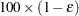% confidence interval for the true probability content of the estimatedth quantile. The default value for the accuracy confidence is 99%, which corresponds to EPS=0.01.

NSAMP=n

specifies the sample size for the simulation. By default,is set based on the values of the target accuracy radiusand accuracy confidence% for an interval for the true probability content of the estimatedth quantile. With the default values for,, and(0.005, 0.01, and 0.05, respectively), NSAMP=12,604 by default.

SEED=number

specifies an integer that is used to start the pseudo-random number generator for the simulation. If you do not specify a seed, or specify a value less than or equal to zero, the seed is by default generated from reading the time of day from the computer’s clock.

specifies that the computational work for the simulation be divided into parallel threads, where the number of threads is the value of the SAS system option CPUCOUNT=. For large simulations (as specified directly using the NSAMP= simoption or indirectly using the ACC= or EPS= simoptions), parallel processing can markedly speed up the computation of adjusted p-values and confidence intervals. However, because the parallel processing has different pseudo-random number streams, the precise results are different from the default ones, which are computed in sequence rather than in parallel. This option overrides the SAS system option THREADS | NOTHREADS.

specifies that the computational work for the simulation be performed in sequence rather than in parallel. NOTHREADS is the default. This option overrides the SAS system option THREADS | NOTHREADS.

If the STEPDOWN option is in effect, the p-values are further adjusted in a step-down fashion. For certain options and data, this adjustment is exact under an iidmodel for the dependent variable, in particular for the following:

• for ADJUST=DUNNETT when the means are uncorrelated

• for ADJUST=TUKEY with STEPDOWN(TYPE=LOGICAL) when the means are balanced and uncorrelated.

The first case is a consequence of the nature of the successive step-down hypotheses for comparisons with a control; the second uses an extension of the maximum studentized range distribution appropriate for partition hypotheses (Royen 1989). Finally, for STEPDOWN(TYPE=FREE), ADJUST=TUKEY employs the Royen (1989) extension in such a way that the resulting p-values are conservative.

ALPHA=number

requests that a t type confidence interval be constructed for each of the LS-means with confidence level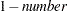. The value of number must be between 0 and 1; the default is 0.05.

AT variable=value
AT (variable-list)=(value-list)
AT MEANS

modifies the values of the covariates that are used in computing LS-means. By default, all covariate effects are set equal to their mean values for computation of standard LS-means. The AT option enables you to assign arbitrary values to the covariates. Additional columns in the output table indicate the values of the covariates.

If there is an effect that contains two or more covariates, the AT option sets the effect equal to the product of the individual means rather than the mean of the product (as with standard LS-means calculations). The AT MEANS option sets covariates equal to their mean values (as with standard LS-means) and incorporates this adjustment to crossproducts of covariates.

As an example, consider the following statements:

```class A;
model Y = A x1 x2 x1*x2;
lsmeans A;
lsmeans A / at means;
lsmeans A / at x1=1.2;
lsmeans A / at (x1 x2)=(1.2 0.3);
```

For the first two LSMEANS statements, the LS-means coefficient for x1 is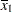(the mean of x1) and for x2 is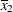(the mean of x2). However, for the first LSMEANS statement, the coefficient for x1*x2 is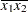, but for the second LSMEANS statement, the coefficient is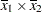. The third LSMEANS statement sets the coefficient for x1 equal to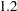and leaves it atfor x2, and the final LSMEANS statement sets these values toand, respectively.

Even if you specify a WEIGHT variable, the unweighted covariate means are used for the covariate coefficients if there is no AT specification. If you specify the AT option, WEIGHT or FREQ variables are taken into account as follows. The weighted covariate means are then used for the covariate coefficients for which no explicit AT values are given, or if you specify AT MEANS. Observations that do not contribute to the analysis because of a missing dependent variable are included in computing the covariate means. Use the E option in conjunction with the AT option to check that the modified LS-means coefficients are the ones you want.

The AT option is disabled if you specify the BYLEVEL option.

BYLEVEL

requests that separate margins be computed for each level of the LSMEANS effect.

The standard LS-means have equal coefficients across classification effects. The BYLEVEL option changes these coefficients to be proportional to the observed margins. This adjustment is reasonable when you want your inferences to apply to a population that is not necessarily balanced but has the margins observed in the input data set. In this case, the resulting LS-means are actually equal to raw means for fixed-effects models and certain balanced random-effects models, but their estimated standard errors account for the covariance structure that you have specified. If a WEIGHT statement is specified, the procedure uses weighted margins to construct the LS-means coefficients.

If the AT option is specified, the BYLEVEL option disables it.

CL

requests that t type confidence limits be constructed for each of the LS-means. The confidence level is 0.95 by default; this can be changed with the ALPHA= option. If you specify an ADJUST= option, then the confidence limits are adjusted for multiplicity. But if you also specify STEPDOWN, then only p-values are step-down adjusted, not the confidence limits.

CORR

displays the estimated correlation matrix of the least squares means as part of the "Least Squares Means" table.

COV

displays the estimated covariance matrix of the least squares means as part of the "Least Squares Means" table.

DF=number

specifies the degrees of freedom for the t test and confidence limits. The default is the denominator degrees of freedom taken from the "Type III Tests" table that corresponds to the LS-means effect. The option is not supported by the procedures that perform chi-square-based inference (GENMOD, LOGISTIC, PHREG and SURVEYLOGISTIC).

DIFF<=difftype>
PDIFF<=difftype>

requests that differences of the LS-means be displayed. You can use one of the following optional difftype values to specify which differences to produce:

ALL

requests all pairwise differences; this is the default.

ANOM

requests differences between each LS-mean and the average LS-mean, as in the analysis of means (Ott 1967). The average is computed as a weighted mean of the LS-means, the weights being inversely proportional to the diagonal entries of thematrix. If LS-means are nonestimable, this design-based weighted mean is replaced with an equally weighted mean. Note that the ANOM procedure in SAS/QC software implements both tables and graphics for the analysis of means with a variety of response types. For one-way designs and normal data with identity link, the DIFF=ANOM computations are equivalent to the results of PROC ANOM. If the LS-means being compared are uncorrelated, exact adjusted p-values and critical values for confidence limits can be computed in the analysis of means; see Nelson (1982, 1991, 1993) and Guirguis and Tobias (2004) in addition to the documentation for the ADJUST=NELSON option.

CONTROL

requests differences with a control, which, by default, is the first level of each of the specified LSMEANS effects. To specify which levels of the effects are the controls, list the quoted formatted values in parentheses after the CONTROL keyword. For example, if the effects A, B, and C are classification variables, each having two levels, 1 and 2, the following LSMEANS statement specifies the (1,2) level of A*B and the (2,1) level of B*C as controls:

```lsmeans A*B B*C / diff=control('1' '2' '2' '1');
```

For multiple effects, the results depend upon the order of the list, and so you should check the output to make sure that the controls are correct.

Two-tailed tests and confidence limits are associated with the CONTROL difftype. For one-tailed results, use either the CONTROLL or CONTROLU difftype.

CONTROLL

tests whether the noncontrol levels are significantly smaller than the control; the upper confidence limits for the control minus the noncontrol levels are considered to be infinity and are displayed as missing.

CONTROLU

tests whether the noncontrol levels are significantly larger than the control; the upper confidence limits for the noncontrol levels minus the control are considered to be infinity and are displayed as missing.

If you want to perform multiple comparison adjustments on the differences of LS-means, you must specify the ADJUST= option.

The differences of the LS-means are displayed in a table titled "Differences of Least Squares Means."

E

requests that thematrix coefficients for the LSMEANS effects be displayed.

EXP

requests exponentiation of the LS-means or LS-mean differences. When you model data with the logit, cumulative logit, or generalized logit link functions, and the estimate represents a log odds ratio or log cumulative odds ratio, the EXP option produces an odds ratio. In proportional hazards model, the exponentiation of the LS-mean differences produces estimates of hazard ratios. If you specify the CL or ALPHA= option, the (adjusted) confidence bounds are also exponentiated.

The EXP option is supported only by PROC PHREG, PROC SURVEYPHREG, the procedures that support generalized linear modeling (GENMOD, LOGISTIC, and SURVEYLOGISTIC), and PROC PLM when it is used to perform statistical analyses on item stores that are created by these procedures.

requests that estimates and their standard errors in the "Least Squares Means" table also be reported on the scale of the mean (the inverse linked scale). This enables you to obtain estimates of predicted probabilities and their standard errors in logistic models, for example. The option is specific to an LSMEANS statement. If you also specify the CL option, the procedure computes confidence intervals for the predicted means by applying the inverse link transform to the confidence limits on the linked (linear) scale. Standard errors on the inverse linked scale are computed by the delta method.

The ILINK option is supported only by the procedures that support generalized linear modeling (GENMOD, LOGISTIC and SURVEYLOGISTIC) and by PROC PLM when it is used to perform statistical analyses on item stores that are created by these procedures.

LINES

presents results of comparisons between all pairs of least squares means by listing the means in descending order and indicating nonsignificant subsets by line segments beside the corresponding LS-means. When all differences have the same variance, these comparison lines are guaranteed to accurately reflect the inferences that are based on the corresponding tests, which are made by comparing the respective p-values to the value of the ALPHA= option (0.05 by default). However, equal variances might not be the case for differences between LS-means. If the variances are not all the same, then the comparison lines might be conservative, in the sense that if you base your inferences on the lines alone, you will detect fewer significant differences than the tests indicate. If there are any such differences, the procedure lists the pairs of means that are inferred to be significantly different by the tests but not by the comparison lines. However, even though the variances in many cases are unequal, they are similar enough that the comparison lines accurately reflect the test inferences.

MEANS|NOMEANS

determines whether to print the least squares means themselves. For most procedure, MEANS is the default behavior. For example, the NOMEANS option is the default for the PHREG procedure. You can then use the MEANS option to produce the table of least squares means, if desired.

ODDSRATIO
OR

requests that LS-mean differences (DIFF, ADJUST= options) are also reported in terms of odds ratios. The ODDSRATIO option is ignored unless you use either the logit, cumulative logit, or generalized logit link function. If you specify the CL or ALPHA= option, confidence intervals for the odds ratios are also computed. These intervals are adjusted for multiplicity when you specify the ADJUST= option.

The ODDSRATIO option is supported only by the procedures that support generalized linear modeling (GENMOD, LOGISTIC and SURVEYLOGISTIC) and by PROC PLM when it is used to perform statistical analyses on item stores created by these procedures.

OBSMARGINS<=OM-data-set>
OM<=OM-data-set>

specifies a potentially different weighting scheme for the computation of LS-means coefficients. The standard LS-means have equal coefficients across classification effects; however, the OM option changes these coefficients to be proportional to those found in the OM-data-set. This adjustment is reasonable when you want your inferences to apply to a population that is not necessarily balanced but has the margins that are observed in OM-data-set.

By default, OM-data-set is the same as the analysis data set. You can optionally specify another data set that describes the population for which you want to make inferences. This data set must contain all model variables except for the dependent variable (which is ignored if it is present). In addition, the levels of all CLASS variables must be the same as those that occur in the analysis data set. Specifying an OM-data-set enables you to construct arbitrarily weighted LS-means.

In computing the observed margins, the procedure uses all observations for which there are no missing or invalid independent variables, including those for which there are missing dependent variables. Also, if you use a WEIGHT statement, the procedure computes weighted margins to construct the LS-means coefficients. If your data are balanced, the LS-means are unchanged by the OM option.

The BYLEVEL option modifies the observed-margins LS-means. Instead of computing the margins across all of the OM-data-set, the procedure computes separate margins for each level of the LSMEANS effect in question. In this case the resulting LS-means are actually equal to raw means for fixed-effects models and certain balanced random-effects models, but their estimated standard errors account for the covariance structure that you have specified.

You can use the E option in conjunction with either the OM or BYLEVEL option to verify that the modified LS-means coefficients are the ones you want. It is possible that the modified LS-means are not estimable when the standard ones are estimable, or vice versa.

PDIFF

is the same as the DIFF option.

PLOT | PLOTS<=plot-request<(options)>>
PLOT | PLOTS<=(plot-request<(options)> <...plot-request<(options)> >)>

requests that graphics related to least squares means be produced via ODS Graphics, provided that the ODS GRAPHICS ON statement has been specified and the plot-request does not conflict with other options in the LSMEANS statement. For general information about ODS Graphics, see Chapter 21, Statistical Graphics Using ODS.

The available options and suboptions are as follows:

ALL

requests that the default plots that correspond to this LSMEANS statement be produced. The default plot depends on the options in the statement.

ANOMPLOT
ANOM

requests an analysis-of-means display in which least squares means are compared to an average least squares mean. Least squares mean ANOM plots are produced only for those model effects that are listed in LSMEANS statements and have options that do not contradict with the display. For example, the following statements produce analysis-of-mean plots for effects A and C:

```lsmeans A / diff=anom plot=anom;
lsmeans B / diff      plot=anom;
lsmeans C /           plot=anom;
```

The DIFF option in the second LSMEANS statement implies all pairwise differences.

BOXPLOT<boxplot-options>

produces box plots of the distribution of the least squares mean or least squares mean differences across a posterior sample. For example, this plot is available in procedures that support a Bayesian analysis through the BAYES statement.

A separate box is generated for each estimable function, and all boxes appear on a single graph by default. You can affect the appearance of the box plot graph with the following options:

ORIENTATION=VERTICAL|HORIZONTAL
ORIENT=VERT|HORIZ

specifies the orientation of the boxes. The default is vertical orientation of the box plots.

NPANELPOS=number

specifies how to break the series of box plots across multiple panels. If the NPANELPOS option is not specified, or if number equals zero, then all box plots are displayed in a single graph; this is the default. If a negative number is specified, then exactly up tonumberof box plots are displayed per panel. If number is positive, then the number of boxes per panel is balanced to achieve small variation in the number of box plots per graph.

CONTROLPLOT

CONTROL

requests a display in which least squares means are visually compared against a reference level. These plots are produced only for statements with options that are compatible with control differences. For example, the following statements produce control plots for effects A and C:

```lsmeans A / diff=control('1') plot=control;
lsmeans B / diff              plot=control;
lsmeans C                     plot=control;
```

The DIFF option in the second LSMEANS statement implies all pairwise differences.

DIFFPLOT<(diffplot-options)>
DIFFOGRAM<(diffplot-options)>
DIFF<(diffplot-options)>

requests a display of all pairwise least squares mean differences and their significance. The display is also known as a "mean-mean scatter plot" when it is based on arithmetic means (Hsu 1996 and Hsu and Peruggia 1994). For each comparison a line segment, centered at the LS-means in the pair, is drawn. The length of the segment corresponds to the projected width of a confidence interval for the least squares mean difference. Segments that fail to cross the 45-degree reference line correspond to significant least squares mean differences.

LS-mean difference plots are produced only for statements with options that are compatible with the display. For example, the following statements request differences against a control level for the A effect, all pairwise differences for the B effect, and the least squares means for the C effect:

```lsmeans A / diff=control('1') plot=diff;
lsmeans B / diff              plot=diff;
lsmeans C                     plot=diff;
```

The DIFF= type in the first statement is incompatible with a display of all pairwise differences.

You can specify the following diffplot-options:

ABS

determines the positioning of the line segments in the plot. This is the default diffplot-options. When the ABS option is in effect, all line segments are shown on the same side of the reference line.

NOABS

determines the positioning of the line segments in the plot. The NOABS option separates comparisons according to the sign of the difference.

CENTER

marks the center point for each comparison. This point corresponds to the intersection of two least squares means.

NOLINE

suppresses the display of the line segments that represent the confidence bounds for the differences of the least squares means. The NOLINES option implies the CENTER option. The default is to draw line segments in the upper portion of the plot area without marking the center point.

DISTPLOT<distplot-options>
DIST<distplot-options>

generates panels of histograms with a kernel density overlaid if the analysis has access to a set of posterior parameter estimates. For example, this plot is available in procedures that support a Bayesian analysis through the BAYES statement. A separate plot in each panel contains the results for each least squares mean or least squares mean differences. You can sepcify the following distplot-options in parentheses:

BOX|NOBOX

controls the display of a horizontal box plot of the estimable function’s distribution across the posterior sample below the graph. The BOX option is enabled by default.

HIST|NOHIST

controls the display of the histogram of the estimable function’s distribution across the posterior sample. The HIST option is enabled by default.

NORMAL|NONORMAL

controls the display of a normal density estimate on the graph. The NONORMAL option is enabled by default.

KERNEL|NOKERNEL

controls the display of a kernel density estimate on the graph. The KERNEL option is enabled by default.

NROWS=number

specifies the highest number of rows in a panel. The default is 3.

NCOLS=number

specifies the highest number of columns in a panel. The default is 3.

UNPACK

unpacks the panel into separate graphics.

MEANPLOT<(meanplot-options)>

requests displays of the least squares means.

The following meanplot-options control the display of the least squares means.

ASCENDING

displays the least squares means in ascending order. This option has no effect if means are displayed in separate plots.

CL

displays upper and lower confidence limits for the least squares means. By default, 95% limits are drawn. You can change the confidence level with the ALPHA= option. Confidence limits are drawn by default if the CL option is specified in the LSMEANS statement.

CLBAND

displays confidence limits as bands. This option implies the JOIN option.

DESCENDING

displays the least squares means in descending order. This option has no effect if means are displayed in separate plots.

requests that means (and confidence limits) be displayed on the inverse linked scale.

JOIN
CONNECT

connects the least squares means with lines. This option is implied by the CLBAND option. If the effect contains nested variables and a SLICEBY= effect contains classification variables that appear as crossed effects, this option is ignored.

SLICEBY=fixed-effect

specifies an effect by which to group the means in a single plot. For example, the following statement requests a plot in which the levels of A are placed on the horizontal axis and the means that belong to the same level of B are joined by lines:

```lsmeans A*B / plot=meanplot(sliceby=b join);
```

Unless the LS-mean effect contains at least two classification variables, the SLICEBY= option has no effect. The SLICEBY= effect does not have to be an effect in your MODEL statement, but it must consist entirely of classification variables.

PLOTBY=fixed-effect

specifies an effect by which to break interaction plots into separate displays. For example, the following statement requests for each level of C one plot of the A*B cell means that are associated with that level of C:

```lsmeans A*B*C / plot=meanplot(sliceby=b plotby=c clband);
```

In each plot, levels of A are displayed on the horizontal axis, and confidence bands are drawn around the means that share the same level of B.

The PLOTBY= option has no effect unless the LS-mean effect contains at least three classification variables. The PLOTBY= effect does not have to be an effect in the MODEL statement, but it must consist entirely of classification variables.

NONE

requests that no plots be produced.

When LS-mean calculations are adjusted for multiplicity by using the ADJUST= option, the plots are adjusted accordingly.

SEED=number

specifies the seed for the sampling-based components of the computations for the LSMEANS statement (for example, chi-bar-square statistics and simulated p-values). number specifies an integer that is used to start the pseudo-random-number generator for the simulation. If you do not specify a seed, or if you specify a value less than or equal to zero, the seed is generated from reading the time of day from the computer clock. Note that there could be multiple LSMEANS statements with SEED= specifications and there could be other statements that can supply a random number seed. Since the procedure has only one random number stream, the initial seed is shown in the SAS log.

SINGULAR=number

tunes the estimability checking. Ifis a vector, define ABS() to be the largest absolute value of the elements of. If ABS(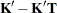) is greater than*number for any row of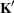in the contrast, then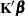is declared nonestimable. Here,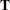is the Hermite form matrix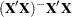, andis ABS(), except when it equals 0, and thenis 1. The value for number must be between 0 and 1; the default is 1E4.

STEPDOWN<(step-down-options)>

requests that multiple comparison adjustments for the p-values of LS-mean differences be further adjusted in a step-down fashion. Step-down methods increase the power of multiple comparisons by taking advantage of the fact that a p-value is never declared significant unless all smaller p-values are also declared significant. The STEPDOWN adjustment combined with ADJUST=BON corresponds to the methods of Holm (1979) "Method 2" of Schaffer (1986); this is the default. Using step-down-adjusted p-values combined with ADJUST=SIMULATE corresponds to the method of Westfall (1997).

If the denominator degrees of freedom are computed by the Kenward-Roger (Kenward and Roger 1997) or Satterthwaite method in a mixed model, then step-down-adjusted p-values are produced only if the ADJDFE=ROW option is in effect.

Also, STEPDOWN affects only p-values, not confidence limits. For ADJUST=SIMULATE, the generalized least squares hybrid approach of Westfall (1997) is used to increase Monte Carlo accuracy.

You can specify the following step-down-options in parentheses:
MAXTIME=n

specifies the time (in seconds) to be spent computing the maximal logically consistent sequential subsets of equality hypotheses for TYPE=LOGICAL. The default is MAXTIME=60. If the MAXTIME value is exceeded, the adjusted tests are not computed. When this occurs, you can try increasing the MAXTIME value. However, note that there are common multiple comparisons problems for which this computation requires a huge amount of time—for example, all pairwise comparisons between more than 10 groups. In such cases, try to use TYPE=FREE (the default) or TYPE=LOGICAL() for small.

REPORT

specifies that a report on the step-down adjustment be displayed, including a listing of the sequential subsets (Westfall 1997) and, for ADJUST=SIMULATE, the step-down simulation results.

TYPE=LOGICAL<(n)>
TYPE=FREE

specifies how step-down adjustment are made. If you specify TYPE=LOGICAL, the step-down adjustments are computed by using maximal logically consistent sequential subsets of equality hypotheses (Shaffer 1986, Westfall 1997). Alternatively, for TYPE=FREE, sequential subsets are computed ignoring logical constraints. The TYPE=FREE results are more conservative than those for TYPE=LOGICAL, but they can be much more efficient to produce for many comparisons. For example, it is not feasible to take logical constraints between all pairwise comparisons of more than 10 groups. For this reason, TYPE=FREE is the default.

However, you can reduce the computational complexity of taking logical constraints into account by limiting the depth of the search tree used to compute them, specifying the optional depth parameter as a numberin parentheses after TYPE=LOGICAL. As with TYPE=FREE, results for TYPE=LOGICAL() are conservative relative to the true TYPE=LOGICAL results. But even for TYPE=LOGICAL() they can be appreciably less conservative than TYPE=FREE, and they are computationally feasible for much larger numbers of comparisons. If you do not specifyor if, the full search tree is used.Previous Page | Next Page | Top of Page